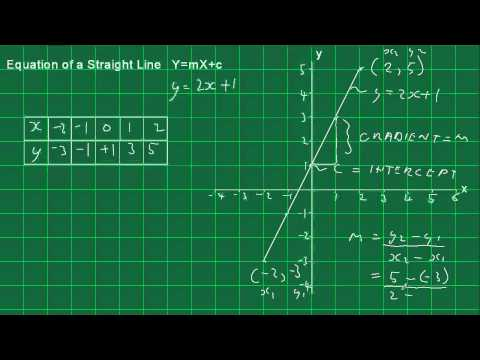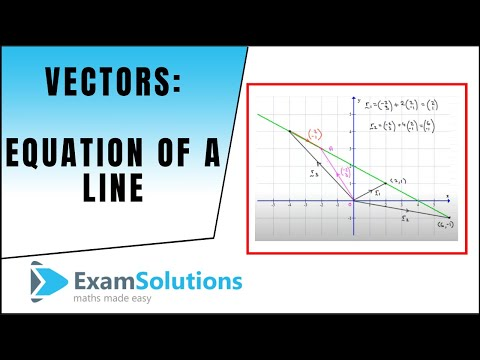## Equation of a Straight Line

Subject: Optional Mathematics#### Overview

An equation of the straight line can be calculated in different ways according to the given condition. This note contents the information about the straight line, horizontal line, slope of a straight line, collinear line, an equation of a straight line, an equation of line straight line and so on.

#### Straight Line

The equation of the straight line can be calculated in different ways according to the given condition. The different conditions of equation of straight line are given below:

#### Horizontal, Vertical and Oblique lines

1. The equation of the straight line parallel to X-axis: Equation of the straight line parallel to X-axis and passing through the point (o, b) cutting at Y-axis i.e. at y = b.
2. The equation of the straight line parallel to Y-axis: Equation of the straight line parallel to Y-axis and passing through the point (a, O) cutting at X-axis is x=a.
3. The oblique line: A line which is neither parallel to x-axis nor parallel to they-axis is called an oblique line.

#### Inclination of a lineWhen the angle is made in a straight line with x-axis making positive direction is said to be the inclination of a line. It is devoted by θ.

#### Slope of Straight line

The tangent of the angle made by the straight line on the positive X-axis is called slope of the straight line. It is denoted by m. If θ be the angle made by the straight line AB on X-axis, then slope AB = m = tanθ

#### Collinear Point

If three or more than three points lies in same straight line then it is said to be collinear points. We can prove the point P, Q, and R collinear by using slope as a slope of PQ = slope of QR =` slope of PR. As the points P, Q, and R in collinear. If the points P, Q, and R lie on the same line the slope of the two line will equal.

#### Intercepts made by a line on the axis

If the line AB cuts the X and Y - axis at the points A (a, O) and B(0, b) respectively. Then the length from the origin to the point of intersection of the line AB and X - axis is called the x -intercept and length from the point of intersection of the line AB and Y - axis are called y - intercept. In the figure , x -intercept OA = a and y -intercept OB = b.

Convention for the signs of intercepts

• If the X-intercepts is measured in right side from the origin then it is considered as positive and measured on the left side from the origin then it is considered as negative.
• If the Y-intercept is measured over the origin then it is considered as positive and measured on the under the origin then it is considered as negative.

#### Equation of a straight line parallel to X-axis

Let AB be a straight line parallel to X-axis. Then the ordinate of every point on the line XY is constant say b.
Let P(x, y) be any point on the lie AB. From P, draw PM perpendicular to X-axis, then MP = y.
∴ y = b, which is required equation of the line AB. The line AB. The line y = b lies above or below the X-axis according to as b is positive or negative.
If b = 0, then the line Ab coincides with X-axis. So, the equation of X-axis is y = 0.

#### Equation of straight line parallel to Y-axis

Let AB be a straight line parallel to Y-axis. Then, the abscissa of every point on the line AB is constant, say a.
Let P(x, y) be any point on the AB. From P, draw PN perpendicular to Y-axis, then NP = x.
∴ x = a, which is required equation of the line AB. The line x = a lies to the right or left of Y-axis according to as a is positive or negative.
If a = 0, then the line Ab coincides with y-axis.

The equation of the straight line in the standard form: There are three standard forms of the equation of the straight line.They are:
Slope - intercept form: If the slope of the straight line m = tanθ and y - intercept (c) are known, the equation of the straight line in slope-intercept form is y = mx + c.

##### Things to remember
1. If the straight line passes through the origin then y - intercept of c = 0. In this case, an equation of the straight line is y = mx.
2. The tangent of the angle made by the straight line on the positive X-axis is called slope of the straight line.
3. When the angle is made in a straight line with x-axis making positive direction is said to be the inclination of a line. It is devoted by θ.
4. If three or more than three points lies in same straight line then it is said to be collinear points.
• It includes every relationship which established among the people.
• There can be more than one community in a society. Community smaller than society.
• It is a network of social relationships which cannot see or touched.
• common interests and common objectives are not necessary for society.
##### Videos for Equation of a Straight Line##### Equation of a Straight Line Introduction##### Vectors - Equation of a Line : Introduction : ExamSolutions

Here, given points (2,5)

The equation of the straight line parallel to y-axis is x=x coordinatesof given points

or, x=2

∴x-2=0Ans.

Here,

given points (5,-2)

The equation of the straight line parallel to y-axis is x=x coordinatesof given points

or, x=5

∴x-5=0Ans.

Given points,(-3,2)

The equation of the straight line parallel to x-axis is y=y-coordinates of the point.

or, y=2

∴ y-2=0.Ans.

Given points,(-3,2)

The equation of the straight line parallel to x-axis is y=y-coordinates of the point.

or, y=-4

∴ y+4=0.Ans.

Here,

The equation of the straight line parallel to x-axis and 4 units above the origin is y=4 and 4 units below the origin is y=-4.

∴The required equation are y-4=0 and y+4=0.Ans.

Here,

Angle on x-axis (θ)=45° and slope of the line(m)=tan 45θ°=1

Equation of straight line passing through origin is y=mx.

or, y=1,x

∴y-x=0.Ans.

Angle on x-axis (θ)=150°

∴ Slope (m)=tanθ=tan150°=-$\frac{1}{√3}$

Using formula,y=mx

y=-$\frac{1}{√3}$x

or,√3y=-x

∴x+√3y=0.Ans.

• Here given, y-intercept(c)=5 units and slope (m)=tanθ=tan60°=√3

Using formula, y=mx+c

y=√3 x+5

∴ y-√3 x-5=0 Ans.

• Here given, y-intercept(c) = 3 units

Slope (m)=tanθ=tan(-60°)=-tan60°= -√3

Using formula y =mx+c

y=-√3 x +3

∴ y+√3 x-3=0.Ans.

• Here given, y-intercept (c)=7 units and slope (m)=tanθ=tan120°=-√3 Using formula y=mx+c y=-√3 x +7 ∴ y+√3 x -7=0.Ans.

Here,

Given equation,x-y=5

or, -y=-x+5...........(i)

Comparing equation (i) with y = mx+c, we get

m=1 and c=-5

∴Slope (m)=1 and y-intercept (c)=-5.Ans.

Here given equation, y=√3 x or y=√3 x+0........(i)

Comparing equation (i) with y=mx +c,we get,

Slope (m)=√3 and y-intercept(c)=0 Ans.

Let x-intercept=y-intercept=a

Equation of straight line is $\frac{x}{a}$+$\frac{y}{b}$=1

$\frac{x}{a}$+$\frac{y}{b}$=1 or, x+y=a......(i)

But equation (i) passes through (5,6).

So, 5+6=a ∴a=11

Putting the value of a in equation (i) we get, required equation x+y=11.Ans.

If x-intercept (a)=a,then y-intercept(b)=-a

Using formula,$\frac{x}{a}$+$\frac{y}{b}$=1

=$\frac{x}{a}$+$\frac{y}{-a}$=1

∴x-y=a..........(i)

But equation (i) passes through(3,2), so

3-2=a ∴a=1

Putting a=1 in equation(i), we get,

Required equation x-y=1.Ans.

Let x-intercept=a then y-intercept (b) =2a

Using formula $\frac{x}{a}$+ $\frac{y}{b}$=1

$\frac{x}{a}$+ $\frac{y}{b}$=1

or, $\frac{2x+y}{2a}$=1

∴2x+y=2a.......(i)

But equation (i) passes through the point (3,4) so,

2× 3 + 4 =2a

or, 6 + 4 = 2a ∴2a=10

Putting the value of 2a in equation (i) we get,

Required equation 2x+y=10 Ans.

Here giiven,

Angle on x-axis is (θ)=45°

Slope (m) = tanθ =tan45°=1

Point (x1y1) =(2,1)

Using formula,

y-y1=m(x-x1) we get,

y-1 =1(x-2)

or,y-1=x-2

or,x-y +1-2=0

∴Required equation x-y-1=0.Ans.

Substituing the point (0,-1) in the equation, we get,

0-(-1)-1=0

or, 1-1=0

∴ 0=0 which is true.

∴ The line x-y-1=0 passes through the point (0,-1).Proved.